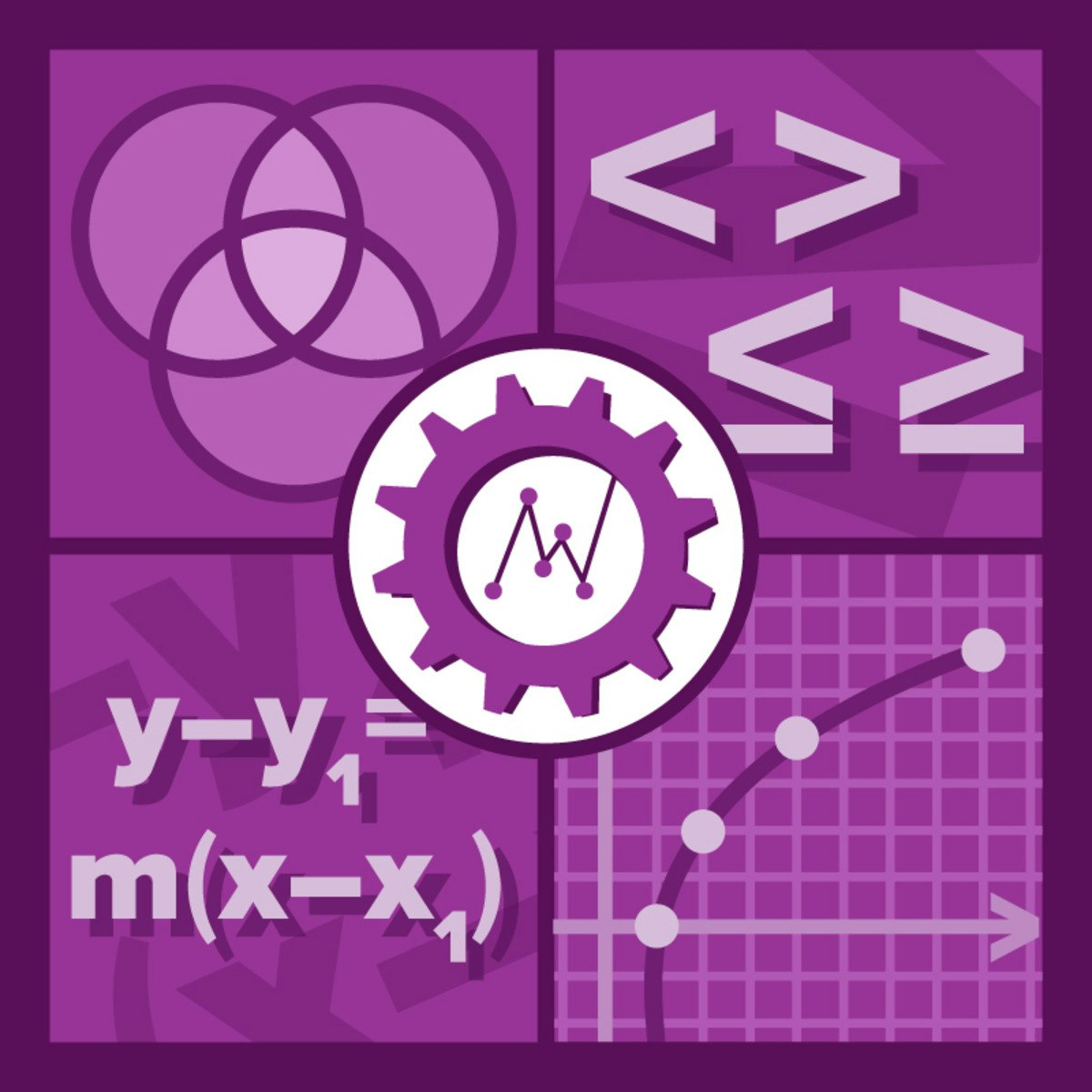Coursera# [100% Off] Data Science Math Skills Free Course Coupon

Duration: 13.0 hours
Expires:

0
0\$ 45.00\$
Get Coupon
Get Coupons instantly by joining our Telegram Channel or Whatsapp Groups

### Description

Data science courses contain math—no avoiding that! This course is designed to teach learners the basic math you will need in order to be successful in almost any data science math course and was created for learners who have basic math skills but may not have taken algebra or pre-calculus. Data Science Math Skills introduces the core math that data science is built upon, with no extra complexity, introducing unfamiliar ideas and math symbols one-at-a-time. Learners who complete this course will master the vocabulary, notation, concepts, and algebra rules that all data scientists must know before moving on to more advanced material. Topics include: ~Set theory, including Venn diagrams ~Properties of the real number line ~Interval notation and algebra with inequalities ~Uses for summation and Sigma notation ~Math on the Cartesian (x,y) plane, slope and distance formulas ~Graphing and describing functions and their inverses on the x-y plane, ~The concept of instantaneous rate of change and tangent lines to a curve ~Exponents, logarithms, and the natural log function. ~Probability theory, including Bayes’ theorem. While this course is intended as a general introduction to the math skills needed for data science, it can be considered a prerequisite for learners interested in the course, "Mastering Data Analysis in Excel," which is part of the Excel to MySQL Data Science Specialization. Learners who master Data Science Math Skills will be fully prepared for success with the more advanced math concepts introduced in "Mastering Data Analysis in Excel."